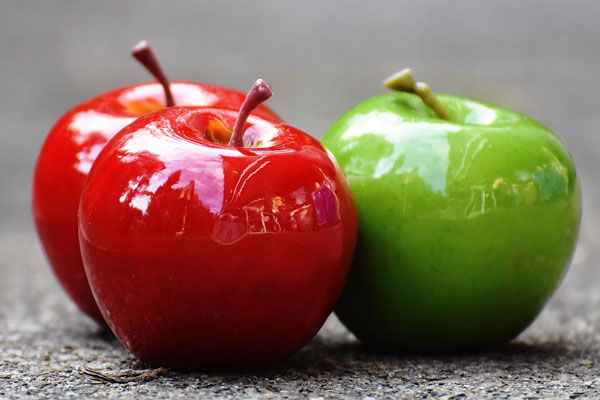# Set

A collection of well-defined objects is called a set.

## Introduction

Each object has some properties naturally. If properties of an object is compared with the properties of other objects, either one or more or all properties may be same. The collection of such objects is called a set.

### Examples

Study the following examples to understand how to define and form a set mathematically.

### ApplesYou are seeing three apples in which an apple is a green apple and the remaining two apples are red colour apples.

All are apples. It is a common property of them. If you consider it and ignore their color, the collection of them is called a set.

If you consider its colour, they cannot form a set. The collection of two red apples form a set and the remaining green apple form another set.

### BallsThere are some footballs in a football stadium and the total footballs is $8$.

All $8$ balls are footballs. Hence, the collection of all eight footballs is a set.

If we consider the size of the footballs, the collection of all eight footballs is not a set because three footballs are in actual size and the other five footballs are in small size. Therefore, the collection $3$ footballs is a set and the collection $5$ footballs is another set.

### CarsObserve the both cars. The properties such as colour, model and everything are same. Hence, the collection of both cars is a set.

The above examples give you an idea about defining a set mathematically according to set theory.

Remember one thing while defining a set; if you want to define a set on the basis of either one or more properties, the objects should have same property or properties commonly. Otherwise, the objects cannot form a set mathematically.

#### Representation

Learn how to represent a set graphically and mathematically in mathematics.

Latest Math Topics
Jun 26, 2023
Jun 23, 2023

###### Math Questions

The math problems with solutions to learn how to solve a problem.

Learn solutions

Practice now

###### Math Videos

The math videos tutorials with visual graphics to learn every concept.

Watch now

###### Subscribe us

Get the latest math updates from the Math Doubts by subscribing us.